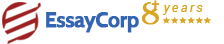WhatsApp
Struggling With Assignments?

We are here to help.

Chat with our expert writers on WhatsApp

for any kind of assignment queries.

Click to chat!
Struggling with Assignments? WhatsApp Us### Question :

Analyze and understand the dataset of different collection of cars and explore the relationship between different variable from a set of eleven variables.Estimation and comparison between the overall regression model and Stepwise Selection procedure. Check all the underlying assumptions for the best fit model and Exploratory data analysis for each of the variable

1. Research question
• Analyze and understand the dataset of different collection of cars and explore the relationship between different variable from a set of eleven variables.
• Estimation and comparison between the overall regression model and Stepwise Selection procedures.
•  Check all the underlining assumptions for the best fit model.
• Exploratory data analysis for each of the variable.
2. Hypothesis
• Testing the significance of Individual Parameters in the model.
• Testing the significance of Overall Regression of the model.
• The model is a good fit for the given data.
• The explanatory variables are independent.
3. Datasets
We are using “mtcars” dataset from R for the purpose of analysis. The data was extracted from the 1974 Motor Trend US magazine, and comprises fuel consumption and 10 aspects of automobile design and performance for 32 automobiles (1973–74 models). This data set consists of 32 observations on the following 11 variables:
 Mpg Miles/(US) gallon Cyl Number of cylinders Disp Displacement (cu.in.) Hp Gross horsepower Drat Rear axle ratio Wt Weight (lb/1000) Qsec 1/4 mile time Vs V/S Am Transmission (0 = automatic, 1 = manual) Gear Number of forward gears Carb Number of carburetors Carb Number of carburetors
Where, Mpg is the dependent variable and cyl,disp,…,carb are independent variables
4. 4. Simple Model Building
1. Fitting a Linear Regression Model
In general the PRF can be any function but for simplicity we restrict ourselves to the class of functions where Y and X1, X2, ... , Xp are related through a linear function of some unknown parameters which leads to linear regression analysis. Let f takes the following form,
Y = β0 + β1X1 + ... + βpXp + ε
Above equation specifies what we call as multiple linear regression model (MLRM) where β0, β1, ... , βp are termed as regression coefficients. We are interested in estimating the PRF which is equivalent to estimate the unknown parameters β0, β1, ... , βp on the basis of a random sample from Y and given values of the independent variables.
Here, we take in our study, “mpg” as dependent variable an rest all other variables viz. “cyl”, “disp”, “hp”, etc. as independent variables X1, X2, ... , Xp.
HIRE EXPERT
Email ID *
Subject
Pages